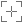# EPSG:7710

## OSGB36 to WGS 84 (9)

### Attributes

Geodetic CRS: OSGB36

Ellipsoid: Airy 1830

Prime meridian: Greenwich

Data source: EPSG

Information source: EPSG

Revision date: 2021-08-19

Scope: (null/copy) Approximation for medium and low accuracy applications assuming equality between plate-fixed static and earth-fixed dynamic CRSs, ignoring static/dynamic CRS differences.

Remarks: Parameter values taken from OSGB36 to ETRS89 (2) (tfm code 7709) assuming that ETRS89 is coincident with WGS 84 within the accuracy of the tfm. Replaces OSGB36 to WGS 84 (7) (tfm code 5339).

Method: NTv2

Description: (0,)United Kingdom (UK) - offshore to boundary of UKCS within 49°45'N to 61°N and 9°W to 2°E; onshore Great Britain (England, Wales and Scotland). Isle of Man onshore.

#### Export

Definition: PROJ.4

`+proj=pipeline +step +proj=axisswap +order=2,1 +step +proj=unitconvert +xy_in=deg +xy_out=rad +step +proj=hgridshift +grids=uk_os_OSTN15_NTv2_OSGBtoETRS.tif +step +proj=unitconvert +xy_in=rad +xy_out=deg +step +proj=axisswap +order=2,1`

Definition: JavaScript (Proj4js)

`proj4.defs("EPSG:7710","+proj=pipeline +step +proj=axisswap +order=2,1 +step +proj=unitconvert +xy_in=deg +xy_out=rad +step +proj=hgridshift +grids=uk_os_OSTN15_NTv2_OSGBtoETRS.tif +step +proj=unitconvert +xy_in=rad +xy_out=deg +step +proj=axisswap +order=2,1");`

Definition: JSON

```{
"\$schema": "https://proj.org/schemas/v0.4/projjson.schema.json",
"type": "Transformation",
"name": "OSGB36 to WGS 84 (9)",
"source_crs": {
"type": "GeographicCRS",
"name": "OSGB36",
"datum": {
"type": "GeodeticReferenceFrame",
"name": "Ordnance Survey of Great Britain 1936",
"ellipsoid": {
"name": "Airy 1830",
"semi_major_axis": 6377563.396,
"inverse_flattening": 299.3249646
}
},
"coordinate_system": {
"subtype": "ellipsoidal",
"axis": [
{
"name": "Geodetic latitude",
"abbreviation": "Lat",
"direction": "north",
"unit": "degree"
},
{
"name": "Geodetic longitude",
"abbreviation": "Lon",
"direction": "east",
"unit": "degree"
}
]
},
"id": {
"authority": "EPSG",
"code": 4277
}
},
"target_crs": {
"type": "GeographicCRS",
"name": "WGS 84",
"datum_ensemble": {
"name": "World Geodetic System 1984 ensemble",
"members": [
{
"name": "World Geodetic System 1984 (Transit)",
"id": {
"authority": "EPSG",
"code": 1166
}
},
{
"name": "World Geodetic System 1984 (G730)",
"id": {
"authority": "EPSG",
"code": 1152
}
},
{
"name": "World Geodetic System 1984 (G873)",
"id": {
"authority": "EPSG",
"code": 1153
}
},
{
"name": "World Geodetic System 1984 (G1150)",
"id": {
"authority": "EPSG",
"code": 1154
}
},
{
"name": "World Geodetic System 1984 (G1674)",
"id": {
"authority": "EPSG",
"code": 1155
}
},
{
"name": "World Geodetic System 1984 (G1762)",
"id": {
"authority": "EPSG",
"code": 1156
}
},
{
"name": "World Geodetic System 1984 (G2139)",
"id": {
"authority": "EPSG",
"code": 1309
}
}
],
"ellipsoid": {
"name": "WGS 84",
"semi_major_axis": 6378137,
"inverse_flattening": 298.257223563
},
"accuracy": "2.0",
"id": {
"authority": "EPSG",
"code": 6326
}
},
"coordinate_system": {
"subtype": "ellipsoidal",
"axis": [
{
"name": "Geodetic latitude",
"abbreviation": "Lat",
"direction": "north",
"unit": "degree"
},
{
"name": "Geodetic longitude",
"abbreviation": "Lon",
"direction": "east",
"unit": "degree"
}
]
},
"id": {
"authority": "EPSG",
"code": 4326
}
},
"method": {
"name": "NTv2",
"id": {
"authority": "EPSG",
"code": 9615
}
},
"parameters": [
{
"name": "Latitude and longitude difference file",
"value": "OSTN15_NTv2_OSGBtoETRS.gsb",
"id": {
"authority": "EPSG",
"code": 8656
}
}
],
"accuracy": "1.0",
"scope": "(null/copy) Approximation for medium and low accuracy applications assuming equality between plate-fixed static and earth-fixed dynamic CRSs, ignoring static/dynamic CRS differences.",
"area": "United Kingdom (UK) - offshore to boundary of UKCS within 49°45'N to 61°N and 9°W to 2°E; onshore Great Britain (England, Wales and Scotland). Isle of Man onshore.",
"bbox": {
"south_latitude": 49.75,
"west_longitude": -9,
"north_latitude": 61.01,
"east_longitude": 2.01
},
"id": {
"authority": "EPSG",
"code": 7710
},
"remarks": "Parameter values taken from OSGB36 to ETRS89 (2) (tfm code 7709) assuming that ETRS89 is coincident with WGS 84 within the accuracy of the tfm. Replaces OSGB36 to WGS 84 (7) (tfm code 5339)."
}
```

Definition: MapServer - MAPfile

```PROJECTION
"proj=pipeline"
"step"
"proj=axisswap"
"order=2,1"
"step"
"proj=unitconvert"
"xy_in=deg"
"step"
"proj=hgridshift"
"grids=uk_os_OSTN15_NTv2_OSGBtoETRS.tif"
"step"
"proj=unitconvert"
"xy_out=deg"
"step"
"proj=axisswap"
"order=2,1"
END```

Definition: Mapnik

```<?xml version="1.0" encoding="utf-8"?>
<Map srs="+proj=pipeline +step +proj=axisswap +order=2,1 +step +proj=unitconvert +xy_in=deg +xy_out=rad +step +proj=hgridshift +grids=uk_os_OSTN15_NTv2_OSGBtoETRS.tif +step +proj=unitconvert +xy_in=rad +xy_out=deg +step +proj=axisswap +order=2,1">
<Layer srs="+proj=pipeline +step +proj=axisswap +order=2,1 +step +proj=unitconvert +xy_in=deg +xy_out=rad +step +proj=hgridshift +grids=uk_os_OSTN15_NTv2_OSGBtoETRS.tif +step +proj=unitconvert +xy_in=rad +xy_out=deg +step +proj=axisswap +order=2,1">
</Layer>
</Map>```

Definition: SQL (PostGIS)

`INSERT into spatial_ref_sys (srid, auth_name, auth_srid, proj4text, srtext) values ( 7710, 'EPSG', 7710, '+proj=pipeline +step +proj=axisswap +order=2,1 +step +proj=unitconvert +xy_in=deg +xy_out=rad +step +proj=hgridshift +grids=uk_os_OSTN15_NTv2_OSGBtoETRS.tif +step +proj=unitconvert +xy_in=rad +xy_out=deg +step +proj=axisswap +order=2,1', 'None');`

Definition: OGC WKT 2 (2019)

`COORDINATEOPERATION["OSGB36 to WGS 84 (9)",VERSION["OGP-UK Gbr15 NT"],SOURCECRS[GEOGCRS["OSGB36",DATUM["Ordnance Survey of Great Britain 1936",ELLIPSOID["Airy 1830",6377563.396,299.3249646,LENGTHUNIT["metre",1]]],PRIMEM["Greenwich",0,ANGLEUNIT["degree",0.0174532925199433]],CS[ellipsoidal,2],AXIS["geodetic latitude (Lat)",north,ORDER,ANGLEUNIT["degree",0.0174532925199433]],AXIS["geodetic longitude (Lon)",east,ORDER,ANGLEUNIT["degree",0.0174532925199433]],ID["EPSG",4277]]],TARGETCRS[GEOGCRS["WGS 84",ENSEMBLE["World Geodetic System 1984 ensemble",MEMBER["World Geodetic System 1984 (Transit)"],MEMBER["World Geodetic System 1984 (G730)"],MEMBER["World Geodetic System 1984 (G873)"],MEMBER["World Geodetic System 1984 (G1150)"],MEMBER["World Geodetic System 1984 (G1674)"],MEMBER["World Geodetic System 1984 (G1762)"],MEMBER["World Geodetic System 1984 (G2139)"],ELLIPSOID["WGS 84",6378137,298.257223563,LENGTHUNIT["metre",1]],ENSEMBLEACCURACY[2.0]],PRIMEM["Greenwich",0,ANGLEUNIT["degree",0.0174532925199433]],CS[ellipsoidal,2],AXIS["geodetic latitude (Lat)",north,ORDER,ANGLEUNIT["degree",0.0174532925199433]],AXIS["geodetic longitude (Lon)",east,ORDER,ANGLEUNIT["degree",0.0174532925199433]],ID["EPSG",4326]]],METHOD["NTv2",ID["EPSG",9615]],PARAMETERFILE["Latitude and longitude difference file","OSTN15_NTv2_OSGBtoETRS.gsb"],OPERATIONACCURACY[1.0],USAGE[SCOPE["(null/copy) Approximation for medium and low accuracy applications assuming equality between plate-fixed static and earth-fixed dynamic CRSs, ignoring static/dynamic CRS differences."],AREA["United Kingdom (UK) - offshore to boundary of UKCS within 49°45'N to 61°N and 9°W to 2°E; onshore Great Britain (England, Wales and Scotland). Isle of Man onshore."],BBOX[49.75,-9,61.01,2.01]],ID["EPSG",7710],REMARK["Parameter values taken from OSGB36 to ETRS89 (2) (tfm code 7709) assuming that ETRS89 is coincident with WGS 84 within the accuracy of the tfm. Replaces OSGB36 to WGS 84 (7) (tfm code 5339)."]]`